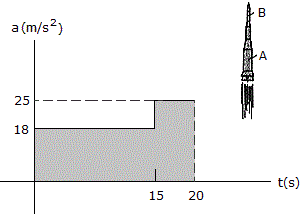# Engineering Mechanics - Kinematics of Particle (KOP) - Discussion

### Discussion :: Kinematics of Particle (KOP) - General Questions (Q.No.2)

2.A two-stage missile is fired vertically from rest with an acceleration as shown in the graph. In 15 s the first stage A burns out and the second stage B ignites. How fast is the rocket moving and how far has it gone at t = 20 s? How fast is the missile moving and how far has it gone at t = 20 s?

 [A]. v = 430 m/s, s = 4.30 km [B]. v = 395 m/s, s = 3.69 km [C]. v = 360 m/s, s = 3.60 km [D]. v = 500 m/s, s = 5.00 km

Explanation:

No answer description available for this question.

 Robin said: (May 26, 2011) Can anyone explain.

 Abhilash Raj said: (Aug 20, 2012) Area=18*15=270m/s=initial velocity(U) V=U+at =270+25*5 =395m/s s=d/t 395=d/20 d=7.9km

 Rajesh Kumar said: (Apr 7, 2013) v = area of shaded region = 18*15+25*5 = 395m/s. s = ut+1/2at*t = 2025m. s1 = u1t1+1/2a1*t1*t1 = 1662.5m. Total distance = s+s1 = 3687.5m = 3.69km.

 Basky said: (Mar 12, 2014) v = Area of shaded region = 18*15+25*5 = 395m/s. s = Average velocity x time. = 395/2 x 20. = 3950. = 3.95m.